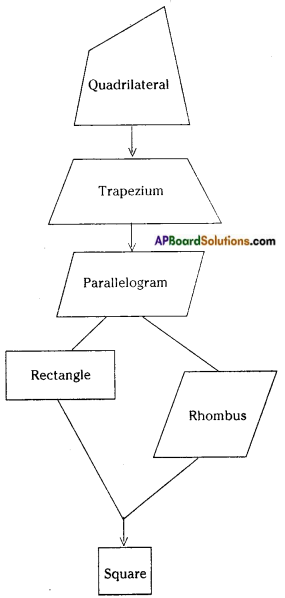Students can go through AP Board 7th Class Maths Notes Chapter 12 Quadrilaterals to understand and remember the concepts easily.

## AP State Board Syllabus 7th Class Maths Notes Chapter 12 Quadrilaterals

→ Quadrilateral: A closed figure bounded by four line segments is called a quadrilateral.
In the figure, ABCD is a quadrilateral.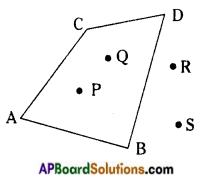→ A quadrilateral divides a plane into three parts.

→ In the figure the points P, Q are in the interior of the quadrilateral. i3r In the figure the points R, S are in the exterior of the quadrilateral.→ In the figure the points A, B, C, D are on the boundary of the quadrilateral.

→ A quadrilateral is said to be a convex quadrilateral if all line segments joining points in the interior of it also lie in its interior completely.
□ BELT is a convex quadrilateral.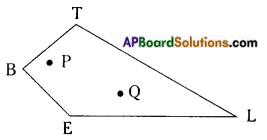→ A quadrilateral is said to be a concave quadrilateral if all line segments joining points in the interior of it do not necessarily lie in its interior completely.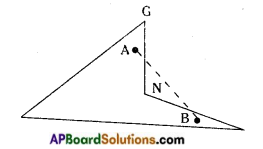In □ RING, the line segment $$\overline{\mathrm{AB}}$$ does not lie completely in its interior, as such the quadrilateral RING is a concave quadrilateral.

→ Sum of the interior angles of a quadrilateral is 360°.
∠A + ∠B + ∠C + ∠D = 360°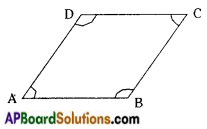→ A quadrilateral in which one pair of opposite sides are parallel is called a trapezium.
In □ ABCD ; AB // CD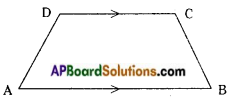→ A kite has four sides. There are exactly two distinct pairs of equal length.KI = KE and IT = ET

→ A quadrilateral in which both pairs of opposite sides are parallel is called a parallelogram. In quadrilateral ABCD,
AB // CD and AD // BC. Hence □ ABCD is a parallelogram.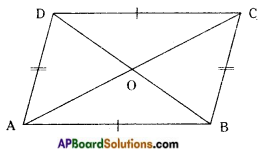→ In a parallelogram,

• Opposite sides are parallel and equal [AB = CD and AD = BC]
• Diagonals bisect each other (AO = OC and BO = OD)
• Opposite angles are equal (∠A = ∠C and ∠B = ∠D)
• Adjacent angles are supplementary (∠A + ∠B = ∠B + ∠C = ∠C + ∠D = ∠D + ∠A = 180°)→ A parallelogram in which adjaœnt sides are equal is called a Rhombus.
AB = BC = CD = DA and hence □ ABCD is a Rhombus.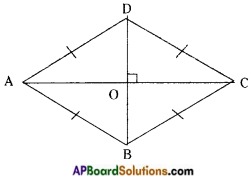In a rhombus diagonals bisect each other at right angles,
(i.e.) AC ⊥ BD and AO = OC, BO = OD

→ A rectangle is a parallelogram with equal angles (OR)
A parallelogram in which one angle is a right angle is called a rectangle.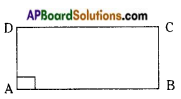In fig. ∠A = ∠B = ∠C = ∠D = 90° and □ ABCD is a rectangle.
In a rectangle the diagonals are equal.
In a rectangle the diagonals bisect each other.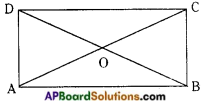(AC = BD and AO = OC; BO = OD)

→ A square is a rectangle with equal adjacent sides.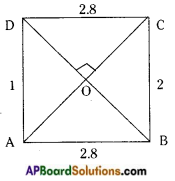In the figure AB = BC = CD = DA
∠A = ∠B = ∠C = ∠D = 90°
In a square the diagonals are equal and bisect at right angles. Also they are equal.
[(AO = OC ; BO = OD), (AC ⊥ BD) and (AC = BD)]Flow chart of family of quadrilaterals